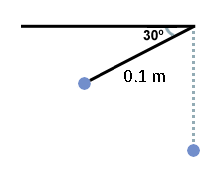# Problem 3

A pendulum with string of length 0.1 m is raised to an angle of $30^\circ$ below the horizontal, as shown below, and then released. What is the velocity (in m/s) of the pendulum when it reaches the bottom ?Use: $g=10 \text{ m/s}^2$.

This is a problem of Energy Tranfers .

×